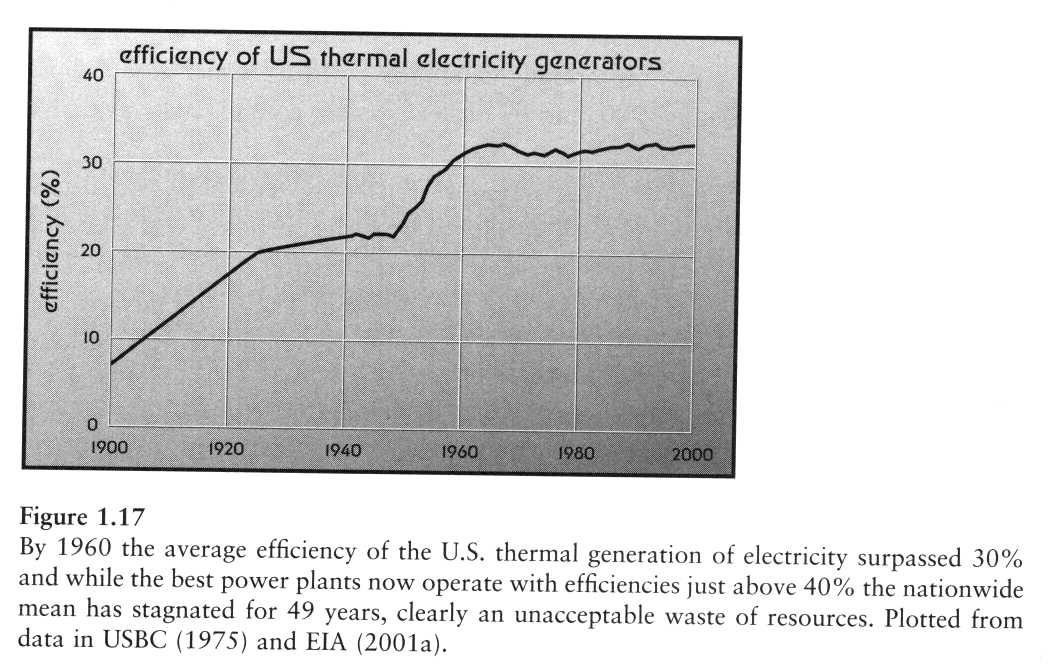Handouts

## How To Think About Efficiency

So, for example, for an engine that converts heat into work, the thing you want is work and the thing you pay for is heat. Hence, the efficiency of an engine is {
$\displaystyle{ \eta = \frac{W}{Q_{\mathrm{in}}} }$
} In a refrigerator, things are different. What you want is heat removed from the "cold space" and what you pay for is work (in the form of electrical power). Hence, the efficiency (or coefficient of performance) of a refrigerator is {
$\displaystyle{ \eta = \frac{Q_{\mathrm{in}}}{W} }$
}

Now a restatement for engineers: A heat engine is a device for converting "heat energy" into useful work.

1. Entropy cannot pile up in such a device; to work in steady state, the net change in the entropy of the device must vanish.
2. Energy cannot pile up in such a device either.
3. The best you can do is to avoid straying from equilibrium, situations in which reversible flows of heat take place.

Carnot's great insight was to think about the procedure without regard to the details of the substances involved, concentrating on the "motive power of fire."

### Heating Processes

When a warmer object is placed in contact with a cooler object, energy flows from the warmer to the cooler object. In the process of losing energy, the entropy of the warmer object declines by

{$\Delta S_{w} = \frac{Q}{T_{w}}$} where {$Q$} is the (heat) energy transferred from the object, and the temperature of the warm object is {$T_w$}. Similarly, the entropy that arrives in the cooler object is given by {
$\displaystyle{ \Delta S_{c} = \frac{Q}{T_{c}} }$
}
The change in the combined entropy of the warmer and cooler object in this process is thus {
$\displaystyle{ \Delta S = \frac{Q}{T_{c}} - \frac{Q}{T_{w}} = Q \frac{T_{w}-T_{c}}{T_{w}T_{c}} \ge 0 }$
}

In the limit that {$T_{w} \to T_{c}$}, no new entropy is created. When {$T_{w} > T_{c}$}, more entropy arrives in the cold object than left the warm one.

## Improvements

There are ways to extract more use from the fuel energy used to produce electricity. One is cogeneration, in which the waste heat is used for some other purpose. Examples include the heating of homes and other buildings and the heating of chemical reactors. Using cogeneration it is possible to use up to 70% of the chemical energy in the fuel. This approach was much discussed in the 1970s in the U.S., but by the late 1990s only about 6% of the total U.S. electricity generating capacity uses cogeneration. By contrast, “Denmark receives 40% and the Netherlands and Finland about 30% of electricity from combined heat and power, mostly from district energy systems.” [Smil, 'Energy at the Crossroads', p. 235.]Figure from Vaclav Smil, Energy at the Crossroads (MIT, Cambridge, 2003) p. 41.
Another approach is combined cycle generation of electricity. This technique combines a gas turbine with more conventional steam turbine generation. Because of material-property limitations, the high-temperature limit for steam generation is about {$655^\circ\mathrm{C} = 928~\mathrm{K}$}, whereas the combustion products tend to have temperatures as high as {$1200^\circ\mathrm{C}$} or more. Because gas turbines can operate at these higher temperatures, but tend to produce exhaust gases that are still very hot ({$450^\circ\mathrm{C} \text{ to } 650^\circ\mathrm{C}$}), the exhaust gases are used to heat the water/steam for the steam turbine. Hence, by combining two generators, it is possible to achieve a much greater temperature difference between the heat source and the cold reservoir, significantly increasing the Carnot efficiency. Taking {$T_{H} = 1200^\circ\mathrm{C} = 1473~\mathrm{K}$} as the high temperature and keeping {$T_{C} = 300~\mathrm{K}$}, we see that {
$\displaystyle{ \eta_{\rm Carnot} = 80\% \qquad \text{and} \qquad \eta_{\rm erp} = 55\% }$
} Hence, converting the existing stock of thermal generation systems to one form or the other of these high-efficiency production methods would significantly decrease the U.S. demand for coal and hydrocarbons, and significantly decrease our carbon emissions.